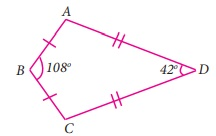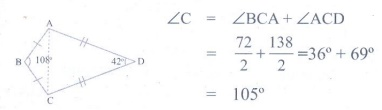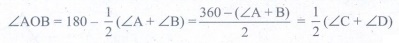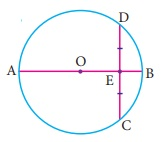Home | | Maths 9th std | Exercise 4.7: Multiple Choice Questions

# Exercise 4.7: Multiple Choice Questions

Maths : Geometry: Book Back, Exercise, Example Numerical Question with Answers, Solution: Exercise 4.7: One Mark Choose the correct Answers

Exercise 4.7

Multiple Choice Questions

1. The exterior angle of a triangle is equal to the sum of two

(1) Exterior angles

(2) Interior opposite angles

(3) Alternate angles

(4) Interior angles

Solution:

Exterior angle = 180° - Interior angle = sum of interior opprsite angle

2. In the quadrilateral ABCD, AB = BC and AD = DC Measure of BCD is(1) 150°

(2) 30°

(3) 105°

(4) 72°

Solution:3. ABCD is a square, diagonals AC and BD meet at O. The number of pairs of congruent triangles with vertex O are(1) 6

(2) 8

(3) 4

(4) 12

4. In the given figure CE || DB then the value of xº is(1) 45°

(2) 30°

(3) 75°

(4) 85°

Solution:

35° + x° + 60° = 180°

x=85°

5. The correct statement out of the following is(1) ΔABC ≈ ΔDEF

(2) ΔABC ≈ ΔDEF

(3) ΔABC ≈ ΔFDE

(4) ΔABC ≈ ΔFED

Solution:

C = ∠D; ∠B=E; ∠A=∠F

6. If the diagonal of a rhombus are equal, then the rhombus is a

(1) Parallelogram but not a rectangle

(2) Rectangle but not a square

(3) Square

(4) Parallelogram but not a square

7. If bisectors of A and B of a quadrilateral ABCD meet at O, then AOB is

(1) C + D

(2) 1/2 (C+ D)

(3) 1/2 C + 1/3 D

(4) 1/3 C + 1/2 D

Solution:8. The interior angle made by the side in a parallelogram is 90° then the parallelogram is a

(1) rhombus

(2) rectangle

(3) trapezium

(4) kite

Solution:

If one angle of a parallelogram is 90, then it is a rectangle.

9. Which of the following statement is correct?

(1) Opposite angles of a parallelogram are not equal.

(2) Adjacent angles of a parallelogram are complementary.

(3) Diagonals of a parallelogram are always equal.

(4) Both pairs of opposite sides of a parallelogram are always equal.

Solution:

Opposite sides of aparallelogram are equal.

[Answer: (4) Both pairs of opposite sides of a parallelogram are always equal.]

10. The angles of the triangle are 3x–40, x+20 and 2x–10 then the value of x is

(1) 40°

(2) 35°

(3) 50°

(4) 45°

Solution:

3x – 40 + x + 20 + 2x – 10 = 180°

6x = 210

x=35°

11. PQ and RS are two equal chords of a circle with centre O such that POQ = 70° , then ORS =

(1) 60°

(2) 70°

(3) 55°

(4) 80°

Solution:12. A chord is at a distance of 15cm from the centre of the circle of radius 25cm. The length of the chord is

(1) 25cm

(2) 20cm

(3) 40cm

(4) 18cm

Solution:13. In the figure, O is the centre of the circle and ACB= 40° then AOB =(1) 80°

(2) 85°

(3) 70°

(4) 65°

Solution:

∠AOB = 2 x ∠ACB = 2 x 40° = 80°

14. In a cyclic quadrilaterals ABCD, A = 4x, C = 2x the value of x is

(1) 30°

(2) 20°

(3) 15°

(4) 25°

Solution:15. In the figure, O is the centre of a circle and diameter AB bisects the chord CD at a point E such that CE=ED=8 cm and EB=4cm. The radius of the circle is C(1) 8cm

(2) 4cm

(3) 6cm

(4) 10cm

Solution:16. In the figure, PQRS and PTVS are two cyclic quadrilaterals, If QRS = 100°, then TVS =(1) 80°

(2) 100°

(3) 70°

(4) 90°

Solution:17. If one angle of a cyclic quadrilateral is 75° , then the opposite angle is

(1) 100°

(2) 105°

(3) 85°

(4) 90°

Solution:

180° – 75° = 105°

18. In the figure, ABCD is a cyclic quadrilateral in which DC produced to E and CF is drawn parallel to AB such that ADC = 80° and ECF = 20°, then BAD = ?(1) 100°

(2) 20°

(3) 120°

(4) 110°

Solution:19. AD is a diameter of a circle and AB is a chord If AD = 30 cm and AB = 24 cm then the distance of AB from the centre of the circle is(1) 10cm

(2) 9cm

(3) 8cm

(3) 6cm

Solution:20. In the given figure, If OP = 17cm, PQ = 30 cm and OS is perpendicular to PQ , then RS is(1) 10cm

(2) 6cm

(3) 7cm

(4) 9cm.

Solution: